Courses

# Polynomials - MCQ Test - 1

## 25 Questions MCQ Test Additional Question Bank | Polynomials - MCQ Test - 1

Description
This mock test of Polynomials - MCQ Test - 1 for Class 9 helps you for every Class 9 entrance exam. This contains 25 Multiple Choice Questions for Class 9 Polynomials - MCQ Test - 1 (mcq) to study with solutions a complete question bank. The solved questions answers in this Polynomials - MCQ Test - 1 quiz give you a good mix of easy questions and tough questions. Class 9 students definitely take this Polynomials - MCQ Test - 1 exercise for a better result in the exam. You can find other Polynomials - MCQ Test - 1 extra questions, long questions & short questions for Class 9 on EduRev as well by searching above.
QUESTION: 1

### If ‘α’ and ‘β’ are the zeroes of a quadratic polynomial ax2+bx+c, then α + β =

Solution:

If α and β are the zeroes of a quadratic polynomial ax2+bx+c, then α+β = -b/a ∵ Sum of the zeroes of a quadratic polynomial ax2+bx+c =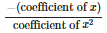QUESTION: 2

### The zeroes of a polynomial x2+5x+6 are

Solution: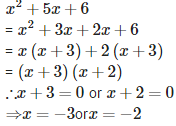QUESTION: 3

### If ‘α’ and ‘β are the zeroes of the polynomial ax2+bx+c, then the value of

Solution: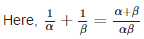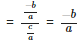QUESTION: 4

If one of the zeroes of the quadratic polynomial 14x2−42k2x−9x is negative of the other, then the value of ‘k’ is

Solution:

Let one zero be ααthen the other zero will be (−α)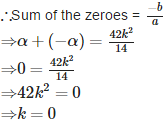QUESTION: 5

A polynomial of degree ____ is called a cubic polynomial.

Solution:

A polynomial of degree 3 is called a cubic polynomial.

QUESTION: 6

The zeroes of the quadratic polynomial x2+9x+20 are

Solution:

x2+9x+20 Splitting the middle term, we get
x2+5x+4x+20
= x(x+5)+4(x+5)
= (x+5)(x+4)
∴x+5 = 0 and x+4 = 0
⇒ x = −5 and x = −4

QUESTION: 7

The zeroes of a polynomial x2+5x−24 are

Solution:

x2+5x−24
= x2+8x−3x−24
= x(x+8)−3(x+8) = (x+8)(x−3)
∴x+8 = 0 or x−3 = 0
⇒ x = −8 or x = 3

QUESTION: 8

If ‘α’ and ‘β’ are the zeroes of the polynomial ax2+bx+c, then the value of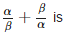Solution: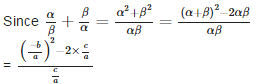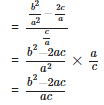QUESTION: 9

The third zero of the polynomial x3+7x2−2x−14, if two of its zeroes are √2 and –√2, is

Solution:

Given: p(x) = x3+7x2−2x−14 If √2 and –√2 are the two zeroes of p(x) then (x−√2) and (x+√2) and also (x−√2) (x+√2) = (x2 - 2) is the factors of p(x).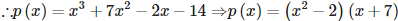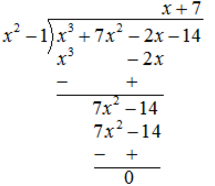Therefore, the third zero is x+7=0 ⇒ x = −7

QUESTION: 10

A polynomial of degree ____ is called a linear polynomial.

Solution:

A polynomial of degree 1 is called a linear polynomial.

QUESTION: 11

A polynomial whose sum and product of zeroes are – 4 and 3 is

Solution:

Here,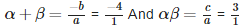On comparing, we get a = 1,b = 4,c = 3 Putting these values in general form of quadratic polynomial ax2+bx+c we get x2+4x+3

QUESTION: 12

The zeroes of a polynomial x2−7x+12 are

Solution:

x2−7x+12
= x2−4x−3x+12
= x(x−4)−3(x−4)
= (x−4)(x−3)
∴x−4 = 0 or x−3 = 0
⇒ x = 4or x = 3

QUESTION: 13

The polynomial 9x2+6x+4 has

Solution:

The polynomial 9x2+6x+4 has no real zeroes because it is not factorizing.

QUESTION: 14

If the product of two of the zeroes of the polynomial 2x3−9x2+13x−6 is 2, then the third zero of the polynomial is

Solution:

Let α,β,γ are the zeroes of the given polynomial. ∴αβ = 2[Given] ∵ αβγ = -d/a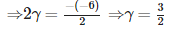QUESTION: 15

A polynomial of degree ‘n’ has

Solution:

A polynomial of degree ‘n’ has at most ‘n’ zeroes because degree of a polynomial is equal to the zeroes of that polynomial only.

QUESTION: 16

A real number ‘k’ is said to be a zero of a polynomial p(x), if p(k) =

Solution:

A real number ‘k’ is said to be a zero of a polynomial p(x), if p(k) is equals to 0.

QUESTION: 17

If the zeroes of a quadratic polynomial ax2 + bx + c, c ≠ 0 are equal, then

Solution:

If the zeroes of a quadratic polynomial ax2+bx+c, c ≠ 0 are equal, then c and a have the same sign always.

QUESTION: 18

The number polynomials having zeroes as – 2 and 5 is

Solution:

The number polynomials having zeroes as –2 and 5 is more than 3. If ‘S’ is the sum and ‘P’ is the product of the zeroes then the corresponding family of quadratic polynomial is given by p(x) = k(x2−Sx+P) where k is any real number. Therefore putting different values of k, we can make more than 3 numbers of polynomials.

QUESTION: 19

If the polynomial 3x3−4x2−17x−k is exactly divisible by x−3, then the value of ‘k’ is

Solution:

If the polynomial 3x3−4x2−17x−k is exactly divisible by x−3, then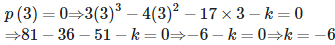QUESTION: 20

The degree of a constant polynomial is

Solution:

The degree of a constant polynomial is 0. e.g. 5 = 5 x 1 = 5×x0

QUESTION: 21

If ‘α’, ‘β’ and ‘γ’ are the zeroes of a cubic polynomial ax3+bx2+cx+d then α + β + γ =

Solution:

If ‘α’, ‘β’ and ‘γ’ are the zeroes of a cubic polynomial ax3+bx2+cx+d, then α + β + γ = -b/a
∵ Sum of the zeroes of a cubic polynomial ax3+bx2+cx+d =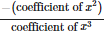QUESTION: 22

If a real number ‘αα ’ is a zero of a polynomial, then ______ is a factor of f(x).

Solution:

If a real number ′α′ is a zero of a polynomial then (x−α) is a factor of that polynomial.

QUESTION: 23

If one of the zeroes of the cubic polynomial x3+ax2+bx+c is – 1, then the product of the other two zeroes is

Solution:

Let α,β,γ are the zeroes of the given polynomial. Given: α = 0 and To find : βγ Since,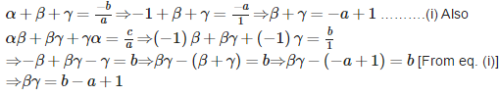QUESTION: 24

On dividing the polynomial 6x3−11x2+7x+5 by 2x−3, the quotient is

Solution: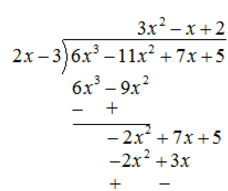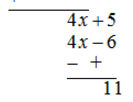QUESTION: 25

The largest power of ‘x’ in p(x) is the _________ of the polynomial.

Solution:

The largest power of ‘x’ in p(x) is the degree of the polynomial.